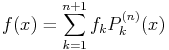2009-06-28 01:58:28 Pang 2.2
Going thru some of my computational physics books, in this case, Tao Pang's intro on the subject. Here's my implementation of problem 2.2 on Lagrangian interpolation done up in F-Script. The formula being programmed is:$tex image$f(x) = \sum_{k=1}^{n+1} f_k P_k^{(n)}(x) where$tex image$P_k^{(n)}(x) = \prod_{j \ne k}^{n+1} \frac{x-x_j}{x_k-x_j}

for a fixed set of known values x for f. The code takes advantage of F-Script's (x,y) point type, which has the setting syntax "x<>y", in which one can later read off, say, the y value, via "myPoint y".

( fscript )
  1  #!/usr/local/bin/fscript  2    3  xValues := { 0.3, 0.5 }.  4  trueFxValues := { 0.328627, 0.520500 }.  5    6  knownDataPoints := {  7      0.0 <> 0.0,       "f1"  8      0.4 <> 0.428392,  9      0.8 <> 0.742101, 10      1.2 <> 0.910314, 11      1.6 <> 0.970348   "f5 = f(n+1)" 12  }. 13   14  weightFunctionP := [ :k :x | 15      weight := 1.0. 16      xk := (knownDataPoints at:k) x. 17      0 to:(knownDataPoints count -1) do:[ :j | 18          (j=k) ifFalse:[ 19              xj := (knownDataPoints at:j) x. 20              weight := weight * (x — xj) / (xk — xj). 21          ]. 22      ]. 23      weight. 24  ]. 25   26  f := [ :x | 27      value := 0.0. 28      0 to:(knownDataPoints count -1) do:[ :k | 29          fk := (knownDataPoints at:k) y. 30          value := value + (fk * (weightFunctionP value:k value:x)). 31      ]. 32      value. 33  ]. 34   35  [ :index | 36      fx := f value:(xValues at:index). 37      trueFx := trueFxValues at:index. 38      accuracy := ((10000 * (trueFx — fx) / trueFx) intValue abs) / 100. "to 2 places" 39   40      xString := (xValues at:index) stringValue. 41      sys out println: 42          '\nf('++xString++') = '++(fx stringValue)++'\n'++ 43          'fTrue('++xString++') = '++(trueFx stringValue)++'\n'++ 44          '  accuracy: '++(accuracy stringValue)++'%'. 45   46  ] value:@(xValues count iota).

which gives the following output:
( terminal )
  1  f(0.3) = 0.3293449013671874  2  fTrue(0.3) = 0.328627  3    accuracy: 0.21%  4    5  f(0.5) = 0.5199387451171874  6  fTrue(0.5) = 0.5205  7    accuracy: 0.1%
• Jeff (Sat, January 7th, 2012, 3:27pm UTC)
Interesting follow-up on this. Now that C has blocks, it looked straight-forward to transliterate this F-Scirpt code into C code. Not only was easy but it's actually better-looking code, despite my love of the former. (Also, it would be trivial in the C version to produce 64-bit results.) The numerical results are slightly different, so I include them at the end.

There is just one gotcha on referencing a C array in a block: you can't, you have to reference a pointer to it. So, one extra line of code to clear that up.

Apart from the few lines of set up at the top, it's almost the same length of code, and you'll note several places where the C code is cleaner, thanks to bracket subscripting, += and *= syntax. The complication, of course, was having to set all variable types and creating a dataPair struct.

See what you think:
( c )
  1  #include <stdio.h>  2  #include <stdlib.h>  3  #include <math.h>  4    5  #define BOUNDS(a) ((sizeof (a))/(sizeof ((a))))  6    7  int main(int argc, const char *argv[]) {  8        9      double xValues[] = { .3, .5 }; 10      double trueFxValues[] = { .328627, .520500 }; 11      struct dataPair { double x,y; }; 12      struct dataPair knownDataPointsRaw[] = { 13          { 0, 0 }, 14          {  .4, .428392 }, 15          {  .8, .742101 }, 16          { 1.2, .910314 }, 17          { 1.6, .970348 } 18      }; 19      struct dataPair *knownDataPoints = knownDataPointsRaw; 20      int knownDataPairCount = BOUNDS( knownDataPointsRaw ); 21       22      double (^weightFunctionP)( int k, double x ) = ^( int k, double x ) { 23          double weight = 1.0; 24          double xk = knownDataPoints[k].x; 25          for( int j=0; j<knownDataPairCount; j++) 26              if (j!=k) { 27                  double xj = knownDataPoints[j].x; 28                  weight *= (x — xj) / (xk — xj); 29              } 30          return weight; 31      }; 32       33      double (^f)( double x ) = ^( double x ) { 34          double value = 0.0; 35          for( int k=0; k<knownDataPairCount; k++ ) { 36              double fk = knownDataPoints[k].y; 37              value += fk * weightFunctionP( k, x ); 38          } 39          return value; 40      }; 41       42      for (int i=0; i<BOUNDS( xValues); i++ ) { 43          double x = xValues[ i ]; 44          double fx = f( xValues[ i ] ); 45          double trueFx = trueFxValues[ i ]; 46          double accuracy = fabs(10000 * (trueFx — fx) / trueFx) / 100; 47          printf( 48              "\nf(%3.1f) [color= %18.16f\n" \ 49              "fTrue(%3.1f) [color= %f\n" \ 50              "  accuracy: %5.2f%%\n\n", 51              x, fx, x, trueFx, accuracy 52          ); 53      } 54       55      return EXIT_SUCCESS; 56  }

( terminal )
  1  f(0.3) = 0.3293449013671874  2  fTrue(0.3) = 0.328627  3    accuracy:  0.22%  4    5    6  f(0.5) = 0.5199387451171875  7  fTrue(0.5) = 0.520500  8    accuracy:  0.11%

• Jeff (Sat, January 7th, 2012, 4:25pm UTC)
Here's a side-by-side image of the two bits of code for quick comparison: